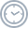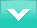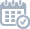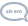# SeminarUpcoming

The Technion Theory Lunch is a seminar run by the theory group at the Department of Computer Science, Technion. The seminar holds weekly meetings in which graduate students and faculty from the Technion and other universities in Israel and abroad present recent results or other interesting topics in theoretical computer science. The seminar is open to faculty, graduate and undergraduate students interested in theory of computing.Wednesdays, 12:30-13:30 (room opens at 12:00)Zoom, Meeting ID: 954 7347 4134, Password: 5-digit Technion Zip Code

If you are interested in giving a talk at the seminar, contact Yuval Filmus.

The seminar’s mailing list is used to announce our weekly meetings, and also to publicize CS theory related events, such as workshops and seminars, in Israel and abroad.

On computing multilinear polynomials using depth four circuits of bounded individual degreeWed, October 28Suryajith Chillara, University of Haifa

The complexity of Iterated Matrix Multiplication is a central theme in Computational Complexity theory, as the problem is closely related to the problem of separating various complexity classes within P. In this talk, we will talk about the complexity of computing an entry in the product of d many n×n generic matrices (denoted IMMn,d) by depth four circuits of bounded individual degree.

We show that for all rna for a fixed constant a, any “syntactic” depth four circuit of bounded individual degree r computing IMMn,d must have size n𝛺(√d) when dnb (for a fixed constant ba). This improves upon a previous result of Kayal, Saha and Tavenas [STOC 2016 & Theory of Computing, 2018] who proved a lower bound of (n/r1.1)𝛺(√d/r) for the same.

This talk assumes no relevant background. We will introduce the model of computation, the motivation to study computation of multilinear polynomials by circuits of bounded individual degree and briefly survey relevant results to this restricted model of computation.

Online talkWed, November 4Ariel Gabizon, Aztec
In a polynomial protocol a prover sends messages that are polynomials, and the verifier is allowed to check polynomial identities between these polynomials. The prover complexity is measured as the sum of degrees of the polynomials sent. The motivation for the definition is to capture prover complexity in zero knowledge proofs systems based on polynomial commitment schemes.
We will present and illustrate this notion; and present an open question on improved protocols for “range proofs” – where given a committed polynomial f, and subset H of the field, we wish to prove f‘s values on H, are in a bounded domain [1,…,M].
We will also attempt to give intuition as to why such range proofs are a crucial component in practical zero-knowledge systems.

(Joint work with Zachary J. Williamson)

Analysis of Two-variable Recurrence Relations with Application to Parameterized ApproximationsWed, November 11Ariel Kulik, Technion

In this paper we introduce randomized branching as a tool for parameterized approximation and develop the mathematical machinery for its analysis. Our algorithms improve the best known running times of parameterized approximation algorithms for Vertex Cover and 3-Hitting Set for a wide range of
approximation ratios. One notable example is a simple parameterized random 1.5-approximation algorithm for Vertex Cover, whose running time of
O*(1.01657k) substantially improves the best known running time of O*(1.0883k) [Brankovic and Fernau, 2013]. For 3-Hitting Set we present a parameterized random \$2\$-approximation algorithm with running time of O*(1.0659k), improving the best known O*(1.29k) algorithm of [Brankovic and Fernau, 2012].

The running times of our algorithms are derived from an asymptotic analysis of a wide class of two-variable recurrence relations. We show an equivalence between these recurrences and a stochastic process, which we analyze using the Method of Types, by introducing an adaptation of Sanov’s theorem to our setting. We believe our novel analysis of recurrence relations, which is of independent interest, is a main contribution of this paper.